How we change what others think, feel, believe and do

Set Theory

DisciplinesArgument > Set Theory

Set Theory is a basic tool of logical argument. It is a simple concept that focuses on understanding how things relate to different categories. It is a common trap to inaccurately assign membership of a group (or set) and consequently make false statements.

Set Theory is best explained using visual overlapping circles, as below. These are called Venn Diagrams. There are also short-hand symbols that are used to write relationships is a mathematical way.

Set Theory and Venn Diagrams are very useful for clarifying and understanding classifications and definitions around the form of 'This is an X, it is not a Y' or 'X and Y have some things in common'.

Membership

A critical first question when identifying something is to categorize it by saying what it is and is not. In set theory, this means identifying to which sets it belongs.

Individual members

In set theory, we say A is a member of B (symbolically: A c B). This means that A has all the attributes of B, that A is a B in all respects.Subsets

Sets can also be nested, so one set is completely within another set (and is hence a subset).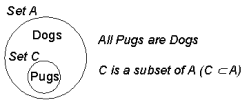Supersets are the inverse of subsets. Thus, if A is a subset of B, then B is a superset of A.

Negation

When an individual member or a subset is outside of a set, the relationship can be described by using a 'not' statement, which is shown symbolically . Although in normal English, we could say 'Cats are not Dogs', in Set Theory, 'not dogs' means everything that is not dogs.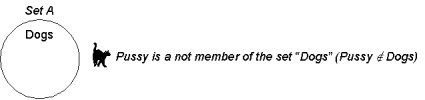Intersection and Union

Above the level of the individual item, the concern is how sets overlap. When two sets may have some members that belong to both sets, we can show this as a Venn diagram with overlapping circles.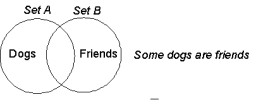Outside the circles are creatures which are neither friends nor dogs.

Intersection

In this diagram, we can look at it in two ways: the overlapping bit and the non-overlapping bit.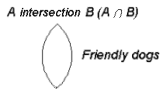The overlapping bit is called the intersection and has members which have attributes of both sets.

In Boolean Algebra, this is an 'and' construct, and may be written A.B, where the dot is shorthand for 'and'.

Union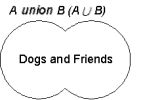When both sets are seen as a single set, this is called the union. In Boolean Algebra, this is an 'or' construct, and may be written as A + B, where the plus sign means 'or' (not the normal arithmetic 'plus').

And...

The remaining pieces are a bit odd, as they are the remaining pieces of each set which is not in the other set. Thus there is 'A and Not B' and 'B and Not A'. The 'not' is often written as a bar above the set name. It also appears as a tilde (~), thus you may also see 'A and ~B' (the tilde is easier to create when typing on a keyboard).In Boolean Algebra, the choice of either of these is called an exclusive or (the intersection is an inclusive or), and is 'A and not B, or not A and B', which also may be written as:

A.~B + ~A.B

And the big
paperback book